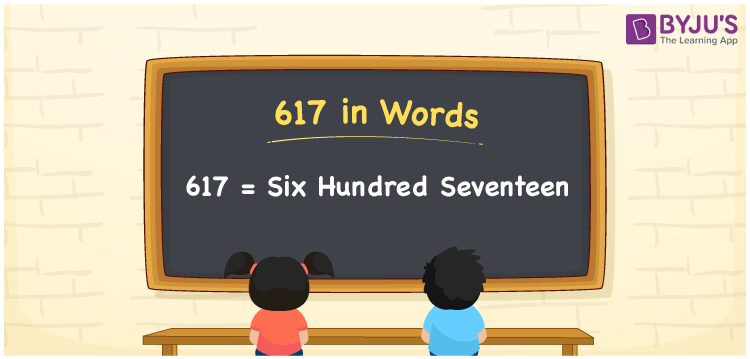# 617 in Words

617 in words is written as Six hundred seventeen. In both the International System of Numerals and the Indian System of Numerals, 617 is written as Six hundred seventeen. The number 617 is a Cardinal Number as it represents some quantity. For example, “617 plates are required”.

 617 in Words Six hundred seventeen Six hundred seventeen in Number 617

## 617 in English Words

We write 617 in English Words using the letters of the English alphabet. Therefore, we read 617 in English as “Six hundred seventeen.”## How to Write 617 in Words?

To write 617 in words, we shall use the place value chart. In the place value chart, write 6 in the hundreds, 1 in the tens, and 7 in the ones, respectively. Now let us make a place value chart to write the number 617 in words.

 Hundreds Tens Ones 6 1 7

Thus, we can write the expanded form as

6 × Hundred + 1 × Ten + 7 × One

= 6 × 100 + 1 × 10 + 7 × 1

= 600 + 10 + 7

= 617

= Six hundred seventeen.

617 is a natural number, the successor of 616 and the predecessor of 618.

617 in words – Six hundred seventeen

• Is 617 an odd number? – Yes
• Is 617 an even number? – No
• Is 617 a perfect square number? – No
• Is 617 a perfect cube number? – No
• Is 617 a prime number? – Yes
• Is 617 a composite number? – No

## Frequently Asked Questions on 617 in Words

Q1

### How to write 617 in words?

617 in words is written as Six hundred seventeen.
Q2

### How to write 617 in the International and Indian System of Numerals?

In both, the system of numerals, 617 in words, is written as Six hundred seventeen.
Q3

### What is the preceding number of 617?

The number that precedes 617 is 616.